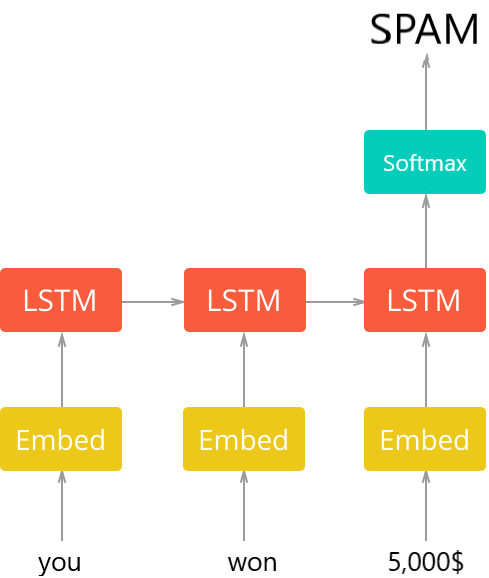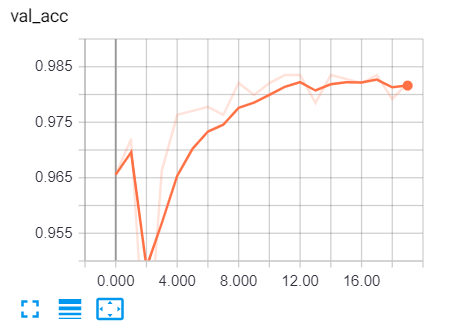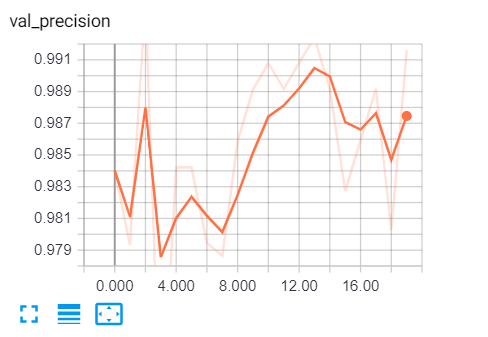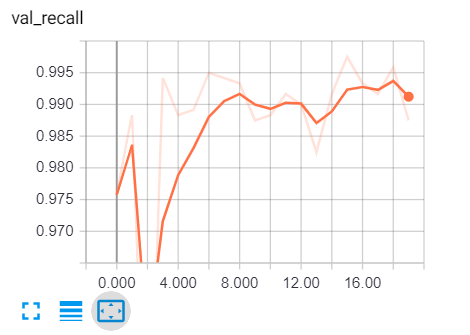# How to Build a Spam Classifier using Keras and TensorFlow in Python

Classifying emails (spam or not spam) with GloVe embedding vectors and RNN/LSTM units using Keras and TensorFlow in Python.
9 min read · Updated jul 2022 · Machine Learning · Natural Language Processing

Disclosure: This post may contain affiliate links, meaning when you click the links and make a purchase, we receive a commission.

Email spam or junk email is unsolicited, unavoidable, and repetitive messages sent in email. Email spam has grown since the early 1990s, and by 2014, it was estimated that it made up around 90% of email messages sent.

Since we all have the problem of spam emails filling our inboxes, in this tutorial, we gonna build a model in Keras that can distinguish between spam and legitimate emails.

Table of content:

## 1. Installing and Importing Dependencies

We first need to install some dependencies:

``pip3 install sklearn tqdm numpy tensorflow``

Now open up an interactive shell or a Jupyter notebook and import:

``````import time
import pickle
import tensorflow as tf
gpus = tf.config.experimental.list_physical_devices('GPU')
if gpus:
# only use GPU memory that we need, not allocate all the GPU memory
tf.config.experimental.set_memory_growth(gpus, enable=True)

import tqdm
import numpy as np
from tensorflow.keras.preprocessing.text import Tokenizer
from tensorflow.keras.utils import to_categorical
from tensorflow.keras.callbacks import ModelCheckpoint, TensorBoard
from sklearn.model_selection import train_test_split
from tensorflow.keras.layers import Embedding, LSTM, Dropout, Dense
from tensorflow.keras.models import Sequential
from tensorflow.keras.metrics import Recall, Precision``````

Let's define some hyper-parameters:

``````SEQUENCE_LENGTH = 100 # the length of all sequences (number of words per sample)
EMBEDDING_SIZE = 100  # Using 100-Dimensional GloVe embedding vectors
TEST_SIZE = 0.25 # ratio of testing set

BATCH_SIZE = 64
EPOCHS = 10 # number of epochs

label2int = {"ham": 0, "spam": 1}
int2label = {0: "ham", 1: "spam"}``````

Don't worry if you are not sure what these parameters mean, we'll talk about them later when we construct our model.

The dataset we gonna use is SMS Spam Collection Dataset, download, extract and put it in a folder called "data", let's define the function that loads it:

``````def load_data():
"""
"""
texts, labels = [], []
with open("data/SMSSpamCollection") as f:
for line in f:
split = line.split()
labels.append(split.strip())
texts.append(' '.join(split[1:]).strip())
return texts, labels``````

The dataset is in a single file, each line corresponds to a data sample, the first word is the label and the rest is the actual email content, that's why we are grabbing labels as split and the content as split[1:].

Calling the function:

``````# load the data

## 3. Preparing the Dataset

Now, we need a way to vectorize the text corpus by turning each text into a sequence of integers, you're now may be wondering why we need to turn the text into a sequence of integers. Well, remember we are going to feed the text into a neural network, a neural network only understands numbers. More precisely, a fixed-length sequence of integers.

But before we do all of that, we need to clean this corpus by removing punctuations, lowercase all characters, etc. Luckily for us, Keras has a built-in class `Tokenizer` from the `tensorflow.keras.preprocessing.text` module, that does all that in few lines of code:

``````# Text tokenization
# vectorizing text, turning each text into sequence of integers
tokenizer = Tokenizer()
tokenizer.fit_on_texts(X)
# lets dump it to a file, so we can use it in testing
pickle.dump(tokenizer, open("results/tokenizer.pickle", "wb"))
# convert to sequence of integers
X = tokenizer.texts_to_sequences(X)``````

Let's try to print the first sample:

``````In : print(X)
[49, 472, 4436, 843, 756, 659, 64, 8, 1328, 87, 123, 352, 1329, 148, 2996, 1330, 67, 58, 4437, 144]``````

A bunch of numbers, each integer corresponds to a word in the vocabulary, that's what the neural network needs anyway. However, the samples don't have the same length, we need a way to have a fixed-length sequence.

As a result, we're using the `pad_sequences()` function from the `tensorflow.keras.preprocessing.sequence` module that pad sequences at the beginning of each sequence with zeros:

``````# convert to numpy arrays
X = np.array(X)
y = np.array(y)
# pad sequences at the beginning of each sequence with 0's
# for example if SEQUENCE_LENGTH=4:
# [[5, 3, 2], [5, 1, 2, 3], [3, 4]]
# will be transformed to:
# [[0, 5, 3, 2], [5, 1, 2, 3], [0, 0, 3, 4]]

As you may remember, we set `SEQUENCE_LENGTH` to 100, in this way, all sequences have a length of 100. Let's print how each sentence is converted to:

``````In : print(X)
[   0    0    0    0    0    0    0    0    0    0    0    0    0    0
0    0    0    0    0    0    0    0    0    0    0    0    0    0
0    0    0    0    0    0    0    0    0    0    0    0    0    0
0    0    0    0    0    0    0    0    0    0    0    0    0    0
0    0    0    0    0    0    0    0    0    0    0    0    0    0
0    0    0    0    0    0    0    0    0    0   49  471 4435  842
755  658   64    8 1327   88  123  351 1328  148 2996 1329   67   58
4436  144]``````

Now our labels are text also, but we gonna make a different approach here, since the labels are only "spam" and "ham", we need to one-hot encode them:

``````# One Hot encoding labels
# [spam, ham, spam, ham, ham] will be converted to:
# [1, 0, 1, 0, 1] and then to:
# [[0, 1], [1, 0], [0, 1], [1, 0], [0, 1]]

y = [ label2int[label] for label in y ]
y = to_categorical(y)``````

We used `keras.utils.to_categorial()` here, which does what its name suggests, let's try to print the first sample of the labels:

``````In : print(y)
[1.0, 0.0]``````

That means the first sample is ham.

Next, let's shuffle and split training and testing data:

``````# split and shuffle
X_train, X_test, y_train, y_test = train_test_split(X, y, test_size=TEST_SIZE, random_state=7)
# print our data shapes
print("X_train.shape:", X_train.shape)
print("X_test.shape:", X_test.shape)
print("y_train.shape:", y_train.shape)
print("y_test.shape:", y_test.shape)``````

Cell output:

``````X_train.shape: (4180, 100)
X_test.shape: (1394, 100)
y_train.shape: (4180, 2)
y_test.shape: (1394, 2)``````

As you can see, we have a total of 4180 training samples and 1494 validation samples.

## 4. Building the Model

Now we are ready to build our model, the general architecture is as shown in the following image:The first layer is a pre-trained embedding layer that maps each word to an N-dimensional vector of real numbers (the EMBEDDING_SIZE corresponds to the size of this vector, in this case, 100). Two words that have similar meanings tend to have very close vectors.

The second layer is a recurrent neural network with LSTM units. Finally, the output layer is 2 neurons each corresponds to "spam" or "ham" with a softmax activation function.

Let's start by writing a function to load the pre-trained embedding vectors:

``````def get_embedding_vectors(tokenizer, dim=100):
embedding_index = {}
with open(f"data/glove.6B.{dim}d.txt", encoding='utf8') as f:
for line in tqdm.tqdm(f, "Reading GloVe"):
values = line.split()
word = values
vectors = np.asarray(values[1:], dtype='float32')
embedding_index[word] = vectors

word_index = tokenizer.word_index
embedding_matrix = np.zeros((len(word_index)+1, dim))
for word, i in word_index.items():
embedding_vector = embedding_index.get(word)
if embedding_vector is not None:
embedding_matrix[i] = embedding_vector

return embedding_matrix``````

Note: In order to run this function properly, you need to download GloVe, extract and put in the "data" folder, we will use the 100-dimensional vectors here.

Let's define the function that builds the model:

``````def get_model(tokenizer, lstm_units):
"""
Constructs the model,
Embedding vectors => LSTM => 2 output Fully-Connected neurons with softmax activation
"""
# get the GloVe embedding vectors
embedding_matrix = get_embedding_vectors(tokenizer)
model = Sequential()
EMBEDDING_SIZE,
weights=[embedding_matrix],
trainable=False,
input_length=SEQUENCE_LENGTH))

# compile as rmsprop optimizer
# aswell as with recall metric
model.compile(optimizer="rmsprop", loss="categorical_crossentropy",
metrics=["accuracy", keras_metrics.precision(), keras_metrics.recall()])
model.summary()
return model``````

The above function constructs the whole model, we loaded the pre-trained embedding vectors to the `Embedding` layer, and set `trainable=False`, this will freeze the embedding weights during the training process.

After we add the RNN layer, we added a 30% dropout chance, this will freeze 30% of neurons in the previous layer in each iteration which will help us reduce overfitting.

Note that accuracy isn't enough for determining whether the model is doing great, that is because this dataset is unbalanced, only a few samples are spam. As a result, we will use precision and recall metrics.

Let's call the function:

``````# constructs the model with 128 LSTM units
model = get_model(tokenizer=tokenizer, lstm_units=128)``````

## 5. Training the Model

We are almost there, we gonna need to train this model with the data we just loaded:

``````# initialize our ModelCheckpoint and TensorBoard callbacks
# model checkpoint for saving best weights
model_checkpoint = ModelCheckpoint("results/spam_classifier_{val_loss:.2f}.h5", save_best_only=True,
verbose=1)
# for better visualization
tensorboard = TensorBoard(f"logs/spam_classifier_{time.time()}")
# train the model
model.fit(X_train, y_train, validation_data=(X_test, y_test),
batch_size=BATCH_SIZE, epochs=EPOCHS,
callbacks=[tensorboard, model_checkpoint],
verbose=1)``````

The training has started:

``````_________________________________________________________________
Layer (type)                 Output Shape              Param #
=================================================================
embedding_1 (Embedding)      (None, 100, 100)          901300
_________________________________________________________________
lstm_1 (LSTM)                (None, 128)               117248
_________________________________________________________________
dropout_1 (Dropout)          (None, 128)               0
_________________________________________________________________
dense_1 (Dense)              (None, 2)                 258
=================================================================
Total params: 1,018,806
Trainable params: 117,506
Non-trainable params: 901,300
_________________________________________________________________
Train on 4180 samples, validate on 1394 samples
Epoch 1/10
66/66 [==============================] - 86s 1s/step - loss: 0.2315 - accuracy: 0.8980 - precision: 0.8980 - recall: 0.8980 - val_loss: 0.1192 - val_accuracy: 0.9555 - val_precision: 0.9555 - val_recall: 0.9555

Epoch 00001: val_loss improved from inf to 0.11920, saving model to results\spam_classifier_0.12.h5
Epoch 2/10
66/66 [==============================] - 87s 1s/step - loss: 0.0824 - accuracy: 0.9726 - precision: 0.9726 - recall: 0.9726 - val_loss: 0.0769 - val_accuracy: 0.9749 - val_precision: 0.9749 - val_recall: 0.9749

Epoch 00002: val_loss improved from 0.11920 to 0.07687, saving model to results\spam_classifier_0.08.h5``````

The training is finished:

``````Epoch 10/10
66/66 [==============================] - 89s 1s/step - loss: 0.0216 - accuracy: 0.9932 - precision: 0.9932 - recall: 0.9932 - val_loss: 0.0546 - val_accuracy: 0.9842 - val_precision: 0.9842 - val_recall: 0.9842

Epoch 00010: val_loss improved from 0.06224 to 0.05463, saving model to results\spam_classifier_0.05.h5``````

## 6. Evaluating the Model

Let's evaluate our model:

``````# get the loss and metrics
result = model.evaluate(X_test, y_test)
# extract those
loss = result
accuracy = result
precision = result
recall = result

print(f"[+] Accuracy: {accuracy*100:.2f}%")
print(f"[+] Precision:   {precision*100:.2f}%")
print(f"[+] Recall:   {recall*100:.2f}%")``````

Output:

``````1394/1394 [==============================] - 1s 569us/step
[+] Accuracy: 98.21%
[+] Precision:   99.16%
[+] Recall:   98.75%``````

Here are what each metric means:

• Accuracy: Percentage of predictions that were correct.
• Recall: Percentage of spam emails that were predicted correctly.
• Precision: Percentage of emails classified as spam that was actually spam.

Great! let's test this out:

``````def get_predictions(text):
sequence = tokenizer.texts_to_sequences([text])
# get the prediction
prediction = model.predict(sequence)
# one-hot encoded vector, revert using np.argmax
return int2label[np.argmax(prediction)]``````

Let's fake a spam email:

``````text = "You won a prize of 1,000\$, click here to claim!"
get_predictions(text)``````

Output:

``spam``

Okay, let's try to be legitimate:

``````text = "Hi man, I was wondering if we can meet tomorrow."
print(get_predictions(text))``````

Output:

``ham``

Awesome! This approach is the current state-of-the-art, try to tune the training and model parameters and see if you can improve it.

To see various metrics during training, we need to go to tensorboard by typing in cmd or terminal:

``tensorboard --logdir="logs"``

Go to the browser and type "localhost:6006" and go to various metrics, here is my result:I encourage you to check the full code.

Finally, if you want to expand your skills in machine learning (or even if you're a beginner), I would suggest you take the Machine Learning and Deep Learning specialization courses. Good luck!

Happy Coding ♥

Sharing is caring!

### Comment panel